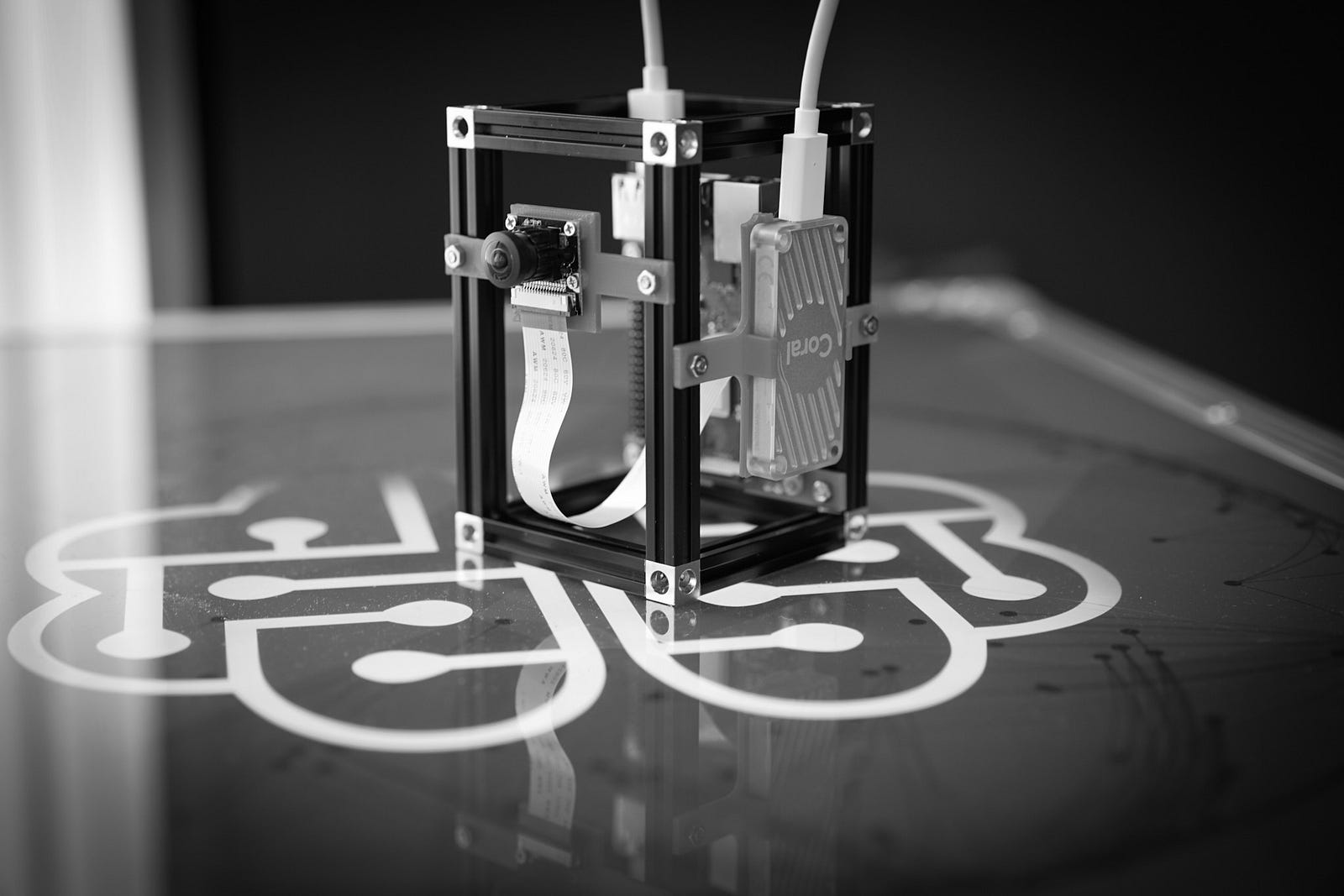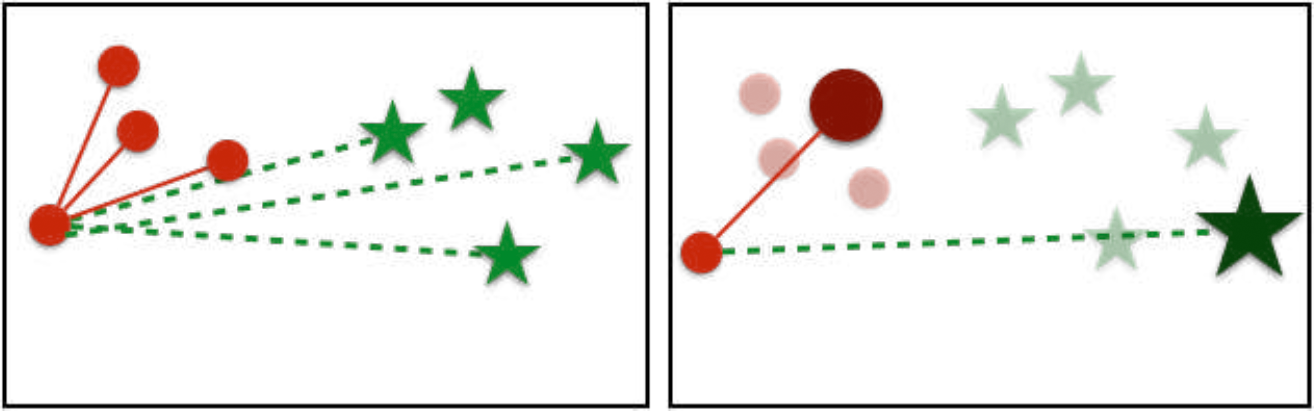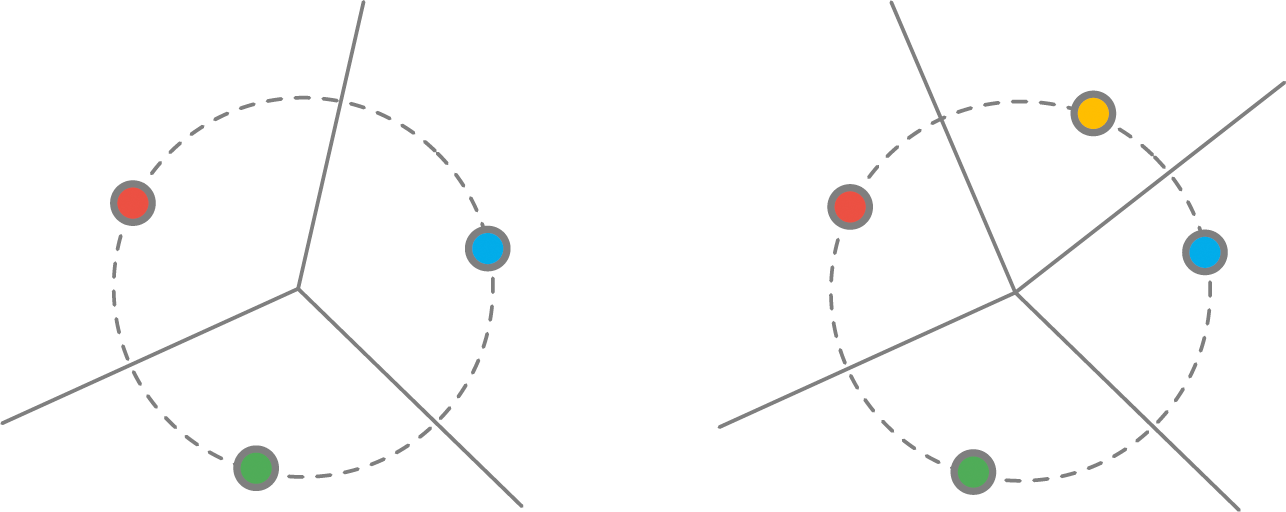# Weight Imprinting on the Coral Edge TPU [Part 1]The Coral Edge TPU is an ideal centerpiece for AI-powered hardware projects like this smart security camera

This article was co-written with my fellow mate and colleague Johannes.

Part 1 describes the theory of Weight Imprinting, Part 2 continues with the implementation of Weight Imprinting on the Coral Edge TPU.

### Motivation

Deep Learning is an amazingly powerful tool that is developing at an insane speed. It is also already part of our daily life when we talk to virtual assistants like Siri or use the Google search.

Most of these services rely on huge data-centers to host the machine learning part. However, for a lot of tasks, it is more appropriate to run the models on the device itself. This paradigm is also called edge-computing. It improves privacy by eliminating the need to transfer sensitive data, e.g. video streams, to third parties and also lets you add machine learning capabilities to devices which are not connected to the internet. Powerful mobile phones like Google’s Pixel 3 already use on-device machine learning to create realistic looking bokeh effects: check out this cool post on Google’s AI blog for more details.

Nowadays, a new wave of low-cost hardware units enables makers to include ML into their own devices. An example of such a device is the recently released Google Coral Edge TPU. It is designed as a dedicated inference unit. While being optimized to run machine learning models to do new predictions, doing the necessary calculations for Backpropagation during training is not possible. So does one still need beefy hardware like GPU workstations? Not necessarily! There are two ways of how existing models can be adapted to new tasks by just using the Coral Edge TPU.

One approach is to use a KNN classifier to learn new classes as described here:

Instead of reading the prediction values from the MobileNet network, we instead take the second to last layer in the neural network and feed it into a KNN (k-nearest neighbors) classifier that allows you to train your own classes.

Another approach is Weight Imprinting. In this article, we not only give you the necessary theoretical understanding of this method but also share an easy to implement project which uses Edge TPU and Weight Imprinting to create a flexible security camera.

Let’s get started!

### The theory behind Weight Imprinting

Before we go deeper into Weight Imprinting itself, we first take a look at a method called “Proxy-Based Embedding Training”, the predecessor of Weight Imprinting. Why? This makes it easier to show the evolution of the technique over time and also offers you a connection between classification and metric learning.

#### Proxy-Based Embedding Training

This method was published in the paper in No Fuss Distance Metric Learning using Proxies. It focuses on metric learning. Basically, the name is the game. Instead of searching or defining a metric that suits a specific problem the best, we try to learn it.

At first, you may be wondering: What is a proxy exactly in the context of Deep Learning. So basically, a proxy is like a mean in k-Means. A vector, that represents a specific set of data points.

One approach to metric learning is the Triplet Loss. A triplet (x,y,z) consists of an anchor point x, a point y that is similar to the anchor point x and a point z that is dissimilar to the anchor point x. The goal of metric learning is to train a network which predicts a small distance d for two similar points and a large distance for dissimilar points. That means for our specific example here: d(x,y) > d(x,z) or equivalent d(x,y) - d(x,z) > 0.

In the case of metric learning, this can be useful because the number of triplets is quite large; in the worst case it can grow cubic with the number of data points.

So let 𝐷 be the set of all data points. The basic idea is to take a subset 𝑃 ⊂ 𝐷, and each element in 𝐷 can be represented by a proxy with an error 𝜖. Instead of forming triplets with all data points in 𝐷, we only use each element in the set of all data points 𝐷 as an anchor point. An example is illustrated in the picture below. In total, there are on both sides eight data points: four dots and four stars. As shown on the left side, forming triplets with all of these results in 48 triplets. By using two proxies we can reduce the number of triplets to only eight.An example of forming triplets with/without proxies. Source: https://arxiv.org/abs/1703.07464

In the end, we are using the Neighborhood Component Analysis (NCA) loss. The NCA loss uses an exponential weighting to reduce the distance between 𝑥 and 𝑦

Using proxies, the NCA simplifies to

#### Weight Imprinting

Now that you know the idea of proxies, we can proceed with Weight Imprinting and the story behind this technique. If you want to read it all, you have to study the paper Low-Shot Learning with Imprinted Weights.

#### Connections between Metric Learning and Softmax Classifiers

The purpose of Weight Imprinting is not to learn a metric but to build a classifier. However, in this case, these two techniques are highly correlated.

Now, let each class have exactly one proxy. Before, we could have multiple proxies for a single class. Let 𝐶 be the set of category labels, then the cardinality |𝐶| is the total number of labels. Let 𝑃 = {𝑝_{1} , 𝑝_{2} , … , 𝑝_{|𝐶|}} be the set of the proxy points. For every data point, you can find the corresponding proxy by the category label 𝑝(𝑥)=𝑝_{𝑐(𝑥)}.

In the following, we assume both the data point 𝑥 as well as the proxy point 𝑝 to be normalized to unit length. Now we have a closer look at the distance between these two points 𝑑(𝑥,𝑝(𝑥)). We use the squared Euclidian distance here, that means:

Because both data points have unit length, we can rewrite it to:

And here comes the simple but effective trick: Minimizing the squared Euclidian distance between two vectors 𝑥 and 𝑝 is equivalent to maximizing the inner product:

which is equal to the cosine similarity since we have unit length vectors.

To close the circle, we insert in the NCA loss of the previous section and add the inner product version:

And if we compare this to the loss of a general softmax classifier, we can see that these two are very similar except the bias term:

This point is crucial. We derived the equation of the classification model by starting with metric learning. Furthermore, this means we can train a classifier and learn the weights using a cross-entropy loss, or we simply choose good proxies. And precisely that is what Weight Imprinting is doing; we set proxies for specific classes.

Now we go back to a general classification network. The fully connected layer at the end is a matrix where each column can be considered as a proxy. Instead of learning the fully connected layer, or in other words learning the weights within the matrix, we can directly construct a matrix with proxies. Each column within the matrix is represented by a proxy that represents a class.Weight Imprinting Scheme. Source: https://arxiv.org/abs/1712.07136

This image above visualizes the underlying network structure. The embeddings are calculated using a pre-trained network. In the paper, they are using an Inception V1. Then the embeddings are normalized to unit length to map them to the unit sphere. Now, these weights are either used for classification or to expand your model by imprinting the weights.

The image below shows two unit spheres in a 2-dimensional space. The colored dots indicate the imprinted weights. On the left side, we have three imprinted weights, on the right side, a fourth one was added. The lines within the circles are the decision boundaries which are exactly between 2 dots. So adding a new Imprinted Weight Vector adds a dot and alters the decision boundaries.Visualization of decision boundaries. Source: https://arxiv.org/abs/1712.07136

If we have more data, this method can be extended by mainly two strategies: average embedding and fine-tuning. Average embedding uses the average over multiple embeddings. However, this only makes sense for unimodal data. For example, cubes with different colors are not unimodal. As stated in the paper, the average over augmented versions of the image does not improve the performance. Probably because the embedded extractor was trained with data augmentation and should be invariant to the transformations. The other option is to fine tune the network. Then the fully connected layer is initialized with the calculated proxies.

From a theoretical standpoint, this method has a few advantages compared to the regular training of a neural network. First and foremost, it can be used for Low-Shot Learning. While training a Neural Network requires a large amount of data, this method often works with just one image per class. Another advantage that you should be aware of is its flexibility. This method allows you to add new classes easily whenever you want or need to.

See Part 2 to learn on how to implement Weight Imprinting on the Coral Edge TPU.